# OECD Gender wage pay gap data analysis 3 - Bootstrapping Confidence Interval and Traditional Method p-value with RThis post is following of the above post.
I will calculate confidence interval.

First, I make bootstrapping confidence interval.

I use R infer package.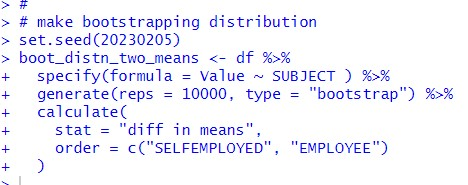I use specify(), generte() and calculate() to make a bootstrapping distribution of SELFEMPLOYED mean and EMPLOYEE mean.

Then, I get confidence interval with get_confidence_interval().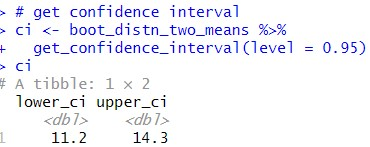95% confidence interval is from 11.2 to 14.3. So, I am 95% confident that the differece of SELFEMPLOYED mean and EMPLOYEE mean is between from 11.2 to 14.3.

Let's visualize that.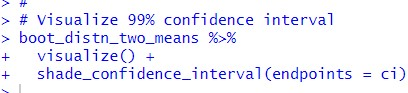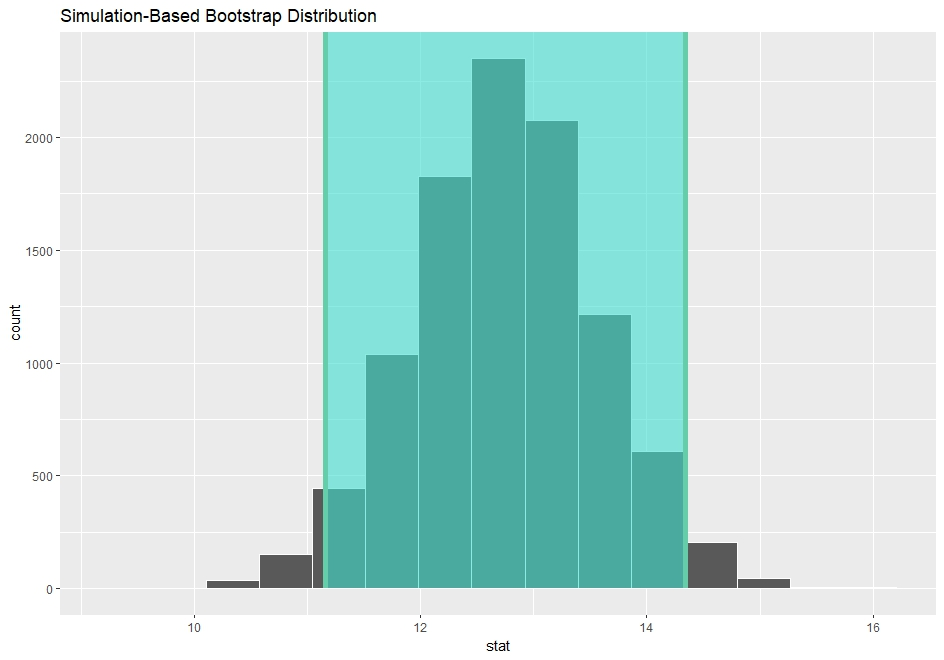Next, I calculate p-value with traditional method(theory based method).
To use traditional method, following two criteria are met.

1. Independent observations

2. Approximately normal

I am not sure that Independent observations criteria, anyway, I will do.

Let's calculate t-static.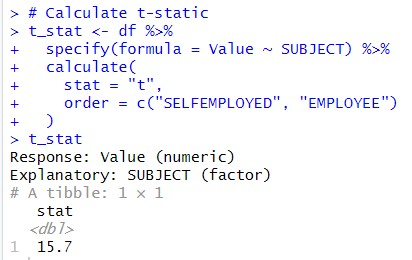t-statistics i1 15.7.

To compute p-value, I need number of observations for each SELFEMPLOYED and EMPLOYEE.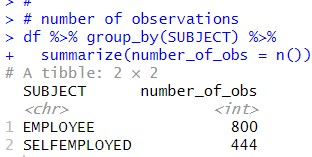EMPLOYEE has 800 and SELFEMPLOYED has 444 observations.
Now, I can calculate p-value.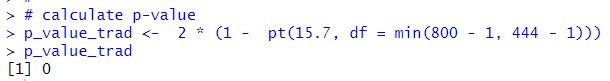I get 0 with traditional method p-value.
In the previous post, I get 0 with simulation based method too.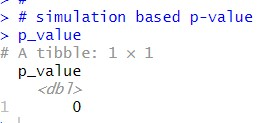That's it! Thank you!

Next post is